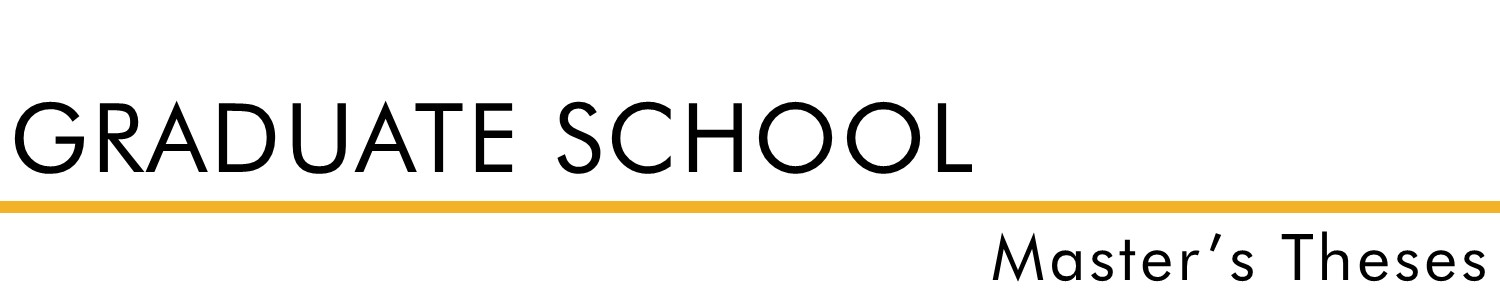#### Document Type

Thesis - campus only access

Summer 1953

#### Degree Name

Master of Science (MS)

#### Department

Math

Dr. Emmet Stopher

#### Abstract

The solving of equations of the third and fourth order is often a very complex problem. It is helpful to know as much about the character of the roots as possible before a solution is attempted. Therefore, t h e problem of this thesis is to derive a set of equations involving the coefficients of the third and fourth order equations that can be used as a test to determine if the equation has any multiple roots. Equations that have real coefficients are the most common type. The problem would become much more complicated if equations which have complex coefficients were included. Therefore, the problem of this thesis is limited to the study of conditions for multiple roots of cubic and quartic equations having real coefficients.

For questions contact ScholarsRepository@fhsu.edu

#### Rights

© 1953 Laurence Dryden

COinS